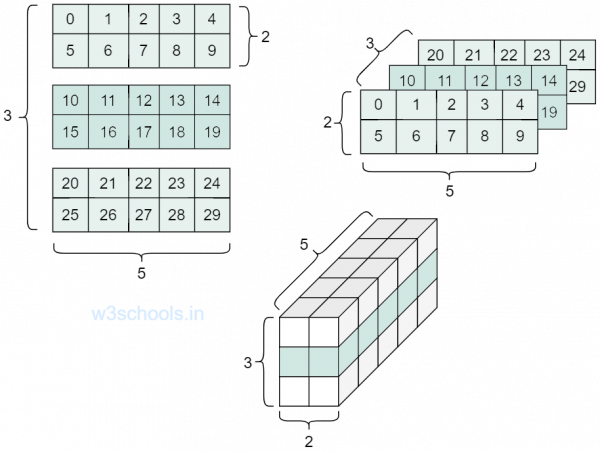## TensorFlow Tutorial Index

As more and more developers started leveraging the TensorFlow framework, it became popular among all artificial intelligence and machine learning engineers. TensorFlow structures are easy to understand because the Tensor we use within it follows a similar data structure to that of NumPy and Pandas library. This tutorial will give you a practical walkthrough of TensorFlow basics and its data structures.

## What Is a Tensor?

A tensor is a generalized vector, and there are matrices of higher dimensions having a uniform type. We can represent this as an array containing different sizes and ranks data. They are fed as input to train the neural network. These tensors can be vectors or arrays (single-dimensional), matrices (two-dimensional), etc. These Tensors can also be three-dimensional, four-dimensional, five-dimensional, etc. All Tensor values are immutable as that of other Python data types like numbers and strings. There is no way to update a tensor's value, element, or content. We can only create a new one.

## What Is a Tensor Data Structure?

A tensor data structure represents various data in a specific Tensor structure having a particular nomenclature. We can determine tensors by defining them through a connecting edge using the flow diagram called Data Flow Graph. Based on a few parameters, we can identify and classify a tensor and its data structure. These are:

1. Tensor Rank: It defines the unit for dimensionality created by tensors. In other words, rank helps determine the number of dimensions a tensor has.
2. Tensor Type: It determines the data type associated with the tenor data structure or elements within the tensors.
3. Tensor Shape:  It determines the number of rows and columns that will be there to form the data structure of the Tensor.
4. Tensor Size: The size defines the total number of items or elements present within a single Tensor unit. It is also responsible for forming the shape of the tensor data structure.

## Dimensions of a Tensor

The tensors in the TensorFlow can be of various dimensions. These are:

### Scalar

These are zero-dimensional (rank 0) tensors and mostly contain one value without any axes.

##### Example Program:
``````import tensorflow as tf

rank_0_val = tf.constant(6)

print(rank_0_val)``````

Output:

`tf.Tensor(6, shape=(), dtype=int32)`

### Vectors

These are one-dimensional (rank 1) tensors and mostly contain one list of values with a single axis.

##### Example Program:
``````import tensorflow as tf

rank_1_val = tf.constant([4.0, 6.0, 8.0])

print(rank_1_val)``````

Output:

`tf.Tensor([4. 6. 8.], shape=(3,), dtype=float32)`

### Matrices

These are two-dimensional (rank 2) tensors and mostly contain a 2D list of values with two axes.

##### Example Program:
``````import tensorflow as tf

rank_2_val = tf.constant([[2, 4],
[3, 6],
[4, 8]], dtype = tf.float16)

print(rank_2_val)``````

Output:

```tf.Tensor(
[[2. 4.]
[3. 6.]
[4. 8.]], shape=(3, 2), dtype=float16)```

### More Axes

There can be tensors with more axes (3, 4, 5, and more). We can create them like this:

##### Example Program:
``````import tensorflow as tf

rank_3_val = tf.constant([
[[1, 2, 3, 4, 5],
[2, 4, 6, 8, 10]],
[[10, 20, 30, 40, 50],
[15, 30, 45, 60, 75]],
[[20, 40, 60, 80, 100],
[25, 50, 75, 100, 125]],])

print(rank_3_val)``````

Output:

```tf.Tensor(
[[[  1   2   3   4   5]
[  2   4   6   8  10]]

[[ 10  20  30  40  50]
[ 15  30  45  60  75]]

[[ 20  40  60  80 100]
[ 25  50  75 100 125]]], shape=(3, 2, 5), dtype=int32)```We can also convert a NumPy array or a Pandas data structure into a Tensor data structure. Here is a code snippet showing its usage:

##### Example Program:
``````import tensorflow as tf
import numpy as np

tf.compat.v1.disable_eager_execution()

m1 = np.array( [(4,4,4), (4,4,4), (4,4,4)], dtype = 'int32')
m2 = np.array( [(2,2,2),(2,2,2),(2,2,2)], dtype = 'int32')
print(m1)
print(m2)

m1 = tf.constant(m1)
m2 = tf.constant(m2)